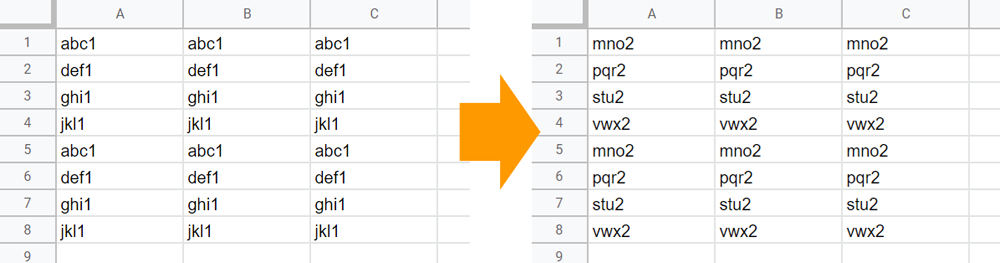# Benchmark: High-Efficiency Finding and Replacing Many Values in Google Spreadsheet with Low Process Cost using Google Apps Script

GistsThis is a sample script for high-efficiency finding and replacing many values in Google Spreadsheet with the low process cost using Google Apps Script.

When the various values are replaced in Google Spreadsheet using Google Apps Script, I’m worried about the process cost. So, in this report, I would like to introduce a sample script for high-efficiency achieving this.

As the result, using a sample situation, when the process cost of the sample script using Sheets API is compared with that of the sample script using Spreadsheet services (SpreadsheetApp), it was found that the above script using Sheets API could reduce the process cost by about 70 % from the script using Spreadsheet service.

## Sample script

function myFunction() {
const sheetId = 0; // Please set the sheet ID.

const replaceData = [
{ from: "abc1", to: "mno2" },
{ from: "def1", to: "pqr2" },
{ from: "ghi1", to: "stu2" },
{ from: "jkl1", to: "vwx2" },
];
const requests = replaceData.map(({ from, to }) => ({
findReplace: {
find: from,
replacement: to,
matchEntireCell: true,
sheetId,
},
}));
}

• When this script is used, the situation at the top image is obtained.

• When the process cost of the above sample script using Sheets API is compared with that of the following sample script using Spreadsheet services (SpreadsheetApp), the above script using Sheets API could reduce the process cost by about 70 % from the script using Spreadsheet service.

function myFunction2() {
const sheetName = "Sheet1";
const replaceData = [
{ from: "abc1", to: "mno2" },
{ from: "def1", to: "pqr2" },
{ from: "ghi1", to: "stu2" },
{ from: "jkl1", to: "vwx2" },
];

const obj = replaceData.reduce(
(o, { from, to }) => ((o[from] = to), o),
{}
);
const sheet =